´

Prescribing a group of automorphisms

The intention is to reduce the incidence matrix to a much smaller matrix so that we can solve the corresponding diophantine system of equations by running a modern implementation of the LLL-algorithm on a workstation or a personal computer. To achieve this goal we use the concept of finite group actions and the prescription of a group of automorphisms (similar to the methods we use in the above mentioned research project on the construction of classical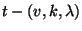-designs). Of course, this prescription is risky since there may be no designs with that particular group of automorphisms, but if there are such designs then it will pay since the number of rows of the incidence matrix, which is the number of-subspaces in, will reduce to the number of orbits of that group, and the same holds for the number of columns. This will bring the construction problem within reach of computers!

An automorphism of a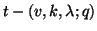-design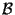is an element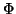of the general linear group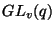which leaves the design invariant, i. e. it maps blocks onto blocks, it permutes the design: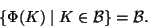A group consisting only of automorphisms of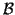is an automorphism group of, the maximal group with this property is called the (full) automorphism group of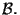The crucial facts are now as simple as this:

2.1 Remarks   If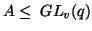is an automorphism group of the-design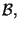then

•consists of full orbits of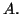We may therefore restrict our attention to the orbits of• The inclusion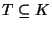of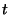-spaces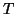in-spaces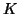is invariant under the action of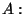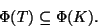• Therefore, for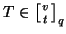and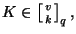the number of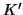in the orbit ofwhich lie aboveis constant on the orbit of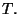• This number is a sum of values of the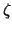-function on the lattice of subspaces of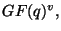and we shall denote it as follows: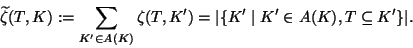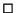This embeds this problem into the theory of group actions on posets (cf. ) and it motivates the introduction of the matrix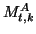, a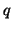-analog of the Kramer-Mesner matrix () for standard combinatorial designs on sets. Its rows (resp. columns) are indicated by the elements(resp.) of a transversal of the set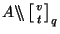of orbits of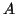on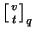(resp. of a transversal of the set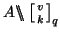of orbits ofon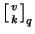), and the entries are the above mentioned values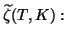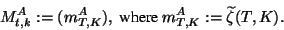The following theorem is now immediate from the above remarks:

2.2 Theorem   The set of-designshaving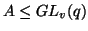as an automorphism group can be obtained from the 0-1-vectors solving the linear system of equations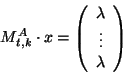in the following way: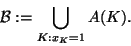This method of generalizing the Kramer-Mesner approach to-analogs of designs was already used in , but the derivation of 2-designs which admit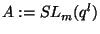which is given there, uses ad hoc methods which cannot be used in the general case. We are therefore going to describe now a general approach that can be used, in principle, in any case. The first step in this direction is the evaluation of transversals of the sets of orbits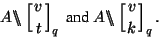For this purpose we can use the Fundamental Lemma of the theory of finite group actions (see e. g. ,) which reads as follows:

2.3   The Fundamental Lemma If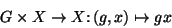denotes a finite group action, and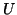a subgroup of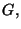then, for each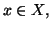the set of orbits ofon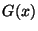can be mapped bijectively onto the set of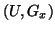-double cosets: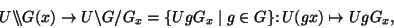where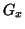means the stabilizer ofin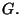In order to apply it we use the fact that the general linear groupis transitive both onand on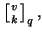and so the following is true:

2.4 Corollary   We can obtain the desired transversals of the orbit sets offrom transversals of sets of double cosets by reverting the following bijections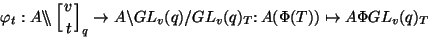and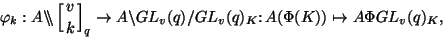whereandare (arbitrary but fixed) elements of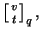respectively, while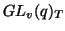and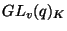denote their stabilizers in the full linear group.Forand, respectively, we can take the subspaces generatedby the, respectivelyfirst unit vectors,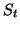and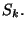The corresponding stabilizers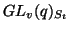and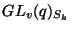consist of the nonsingular matrices with a box of zeros of size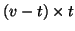and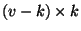in the lower left hand corner.

All what remains is the determination of transversals of the sets of double cosets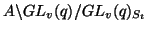and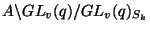. For this purpose we use a generalization of the ladder game introduced by B. Schmalz in ,, in order to construct designs on sets.

Back to the title page

University of Bayreuth -